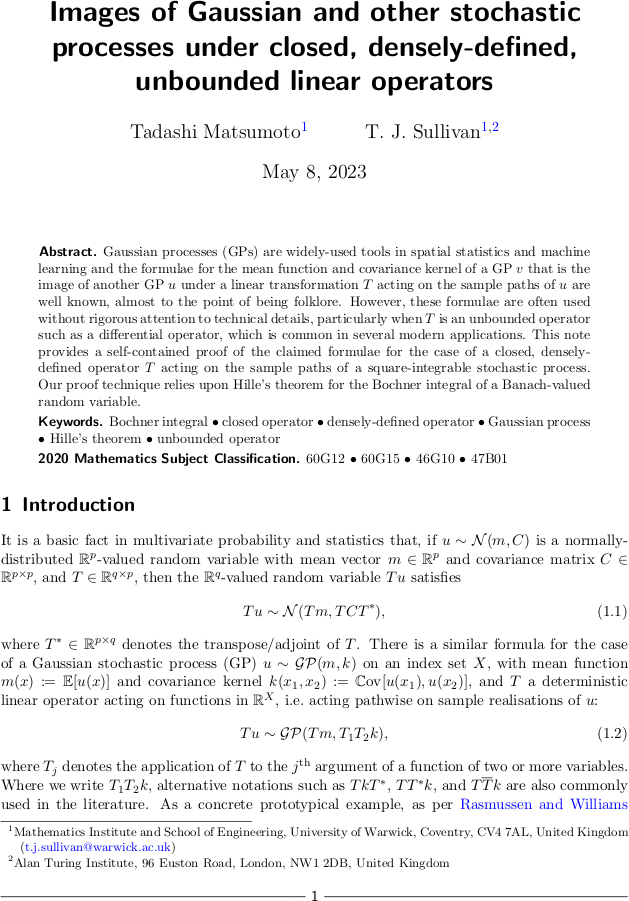# Tim Sullivan

### #matsumoto

Clear Search### Unbounded images of Gaussian and other stochastic processes

Tadashi Matsumoto and I have just uploaded a preprint of our note “Images of Gaussian and other stochastic processes under closed, densely-defined, unbounded linear operators” to the arXiv.

The purpose of this note is to provide a self-contained rigorous proof of the well-known formula for the mean and covariance function of a stochastic process — in particular, a Gaussian process — when it is acted upon by an unbounded linear operator such as an ordinary or partial differential operator, as used in probabilistic approaches to the solution of ODEs and PDEs. This result is easy to establish in the case of a bounded operator, but the unbounded case requires a careful application of Hille's theorem for the Bochner integral of a Banach-valued random variable.

Abstract. Gaussian processes (GPs) are widely-used tools in spatial statistics and machine learning and the formulae for the mean function and covariance kernel of a GP $$v$$ that is the image of another GP $$u$$ under a linear transformation $$T$$ acting on the sample paths of $$u$$ are well known, almost to the point of being folklore. However, these formulae are often used without rigorous attention to technical details, particularly when $$T$$ is an unbounded operator such as a differential operator, which is common in several modern applications. This note provides a self-contained proof of the claimed formulae for the case of a closed, densely-defined operator $$T$$ acting on the sample paths of a square-integrable stochastic process. Our proof technique relies upon Hille's theorem for the Bochner integral of a Banach-valued random variable.

Published on Monday 8 May 2023 at 13:00 UTC #preprint #prob-num #gp #matsumoto